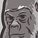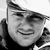# App Development

Discussion board where members can learn more about Qlik Sense App Development and Usage.

Announcements
QlikWorld Online 2021, May 10-12: Our Free, Virtual, Global Event REGISTER TODAY
cancel
Showing results for
Search instead for
Did you mean:Contributor II

## Multiple If Conditions in a variable with multiple selection criteria

Hi,

I'm trying to make my app more "ergonomic" with the use of buttons and make the set analysis still "simple" (not to make the if in the set analysis and being redundant).

The first variable vSelectType can be 4 different values Jaune,Vert,Presta and All. Buttons will define the value of vSelectType.

Then in a second variable vType, i try to make a multiple if conditions that will change the selection criteria in my set analysis like :

if(\$(vSelectType)='Jaune',[Article Vte Famille]={'Bananes'},[Fournisseur Lot Type]=-{'P'},[Fact Ligne Couleur]={'Jaune'},[Fact Type Vente]={'BANANES'},

if(\$(vSelectType)='Vert',[Article Vte Famille]={'Bananes'},[Fournisseur Lot Type]=-{'P'},[Fact Ligne Couleur]={'Vert'},[Fact Type Vente]={'BANANES'},

if(\$(vSelectType)='Presta',[Article Vte Famille]={'Bananes'},[Fact Type Vente]=-{'BANANES'},

if(\$(vSelectType)='All',[Article Vte Famille]={'Bananes'}))))

The set analysis is : sum({\$<\$(vType)>}[Marge Pds Net])

I think the problem is that Qlik think that the , is part of the if and isn't part of the string to replace, when vSelectType changes.

I tried :

'[Article Vte Famille]={'Bananes'},[Fournisseur Lot Type]=-{'P'},[Fact Ligne Couleur]={'Jaune'},[Fact Type Vente]={'BANANES'}'

"[Article Vte Famille]={'Bananes'},[Fournisseur Lot Type]=-{'P'},[Fact Ligne Couleur]={'Jaune'},[Fact Type Vente]={'BANANES'}"

text([Article Vte Famille]={'Bananes'},[Fournisseur Lot Type]=-{'P'},[Fact Ligne Couleur]={'Jaune'},[Fact Type Vente]={'BANANES'})

It's not working

Anybody has a idea ?

Thank you,

Max

Labels (6)

• ### variable

2 Solutions

Accepted SolutionsMVP

May be try this

``````If(vSelectType = 'Jaune', '[Article Vte Famille] = {[Bananes]}, [Fournisseur Lot Type] =- {[P]}, [Fact Ligne Couleur] = {[Jaune]}, [Fact Type Vente] = {[BANANES]}',
If(vSelectType = 'Vert', '[Article Vte Famille] = {[Bananes]}, [Fournisseur Lot Type] =- {[P]}, [Fact Ligne Couleur] = {[Vert]}, [Fact Type Vente] = {[BANANES]}',
If(vSelectType = 'Presta', '[Article Vte Famille] = {[Bananes]}, [Fact Type Vente] =- {[BANANES]}',
If(vSelectType = 'All', '[Article Vte Famille] = {[Bananes]}'))))``````MVP

Can you try this

``Sum({\$<\$(=\$(vType))>} [Marge Pds Net])``

6 RepliesPartner

Have you tried

sum({\$<\$(=vType)>}[Marge Pds Net])

OR

sum({\$<'\$(=vType)'>}[Marge Pds Net])

It will be easier to help if you could create a sample app where you have some sample data and your variables and expressions.

Plees ekskuse my Swenglish and or Norweglish spelling misstakesMVP

May be try this

``````If(vSelectType = 'Jaune', '[Article Vte Famille] = {[Bananes]}, [Fournisseur Lot Type] =- {[P]}, [Fact Ligne Couleur] = {[Jaune]}, [Fact Type Vente] = {[BANANES]}',
If(vSelectType = 'Vert', '[Article Vte Famille] = {[Bananes]}, [Fournisseur Lot Type] =- {[P]}, [Fact Ligne Couleur] = {[Vert]}, [Fact Type Vente] = {[BANANES]}',
If(vSelectType = 'Presta', '[Article Vte Famille] = {[Bananes]}, [Fact Type Vente] =- {[BANANES]}',
If(vSelectType = 'All', '[Article Vte Famille] = {[Bananes]}'))))``````Contributor II
Author

Hi Sunny_Talwar,

Thank you for your answer,

I removed the \$() in the if(vSelectType='Jaune', etc... and put [] around criteria and the if variable is now working !

But I still have problem with the synthax of the primary set analysis : sum({\$<\$(vType)>}[Marge Pds Net])

I already tried with the new if :

sum({\$<\$(vType)>}[Marge Pds Net])

sum({\$<'\$(vType)'>}[Marge Pds Net])

sum({\$<'\$(=vType)'>}[Marge Pds Net])

sum({\$<\$(=vType)>}[Marge Pds Net])

Not workingContributor II
Author

Hi Vegar,

I tried with the new if of Sunny_Talwar :

sum({\$<\$(vType)>}[Marge Pds Net])

sum({\$<'\$(vType)'>}[Marge Pds Net])

sum({\$<'\$(=vType)'>}[Marge Pds Net])

sum({\$<\$(=vType)>}[Marge Pds Net])

Not workingMVP

Can you try this

``Sum({\$<\$(=\$(vType))>} [Marge Pds Net])``Contributor II
Author

It's working perfectly thank you !!